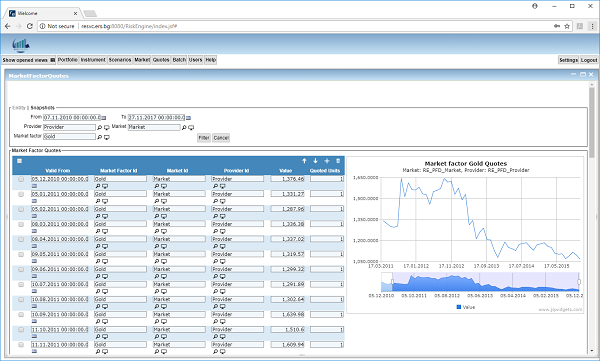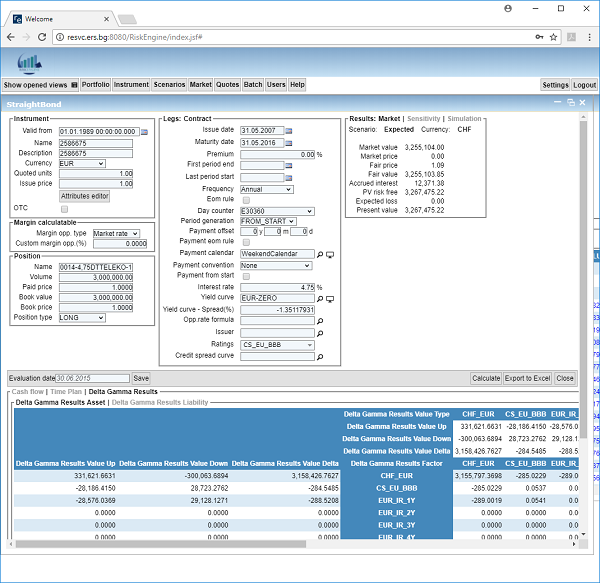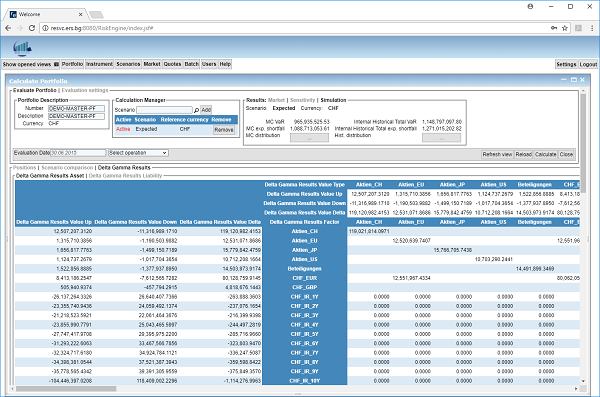# Products

## SWISS SOLVENCY TEST (SST) IN RISK ENGINE

As RE provides more advanced calculations than is required by Swiss Solvency Test (SST), the implementation / calculation of SST is a matter of configuration. The necessary configuration includes:

• Configuration of market factors that take part in the SST market universe.
• Import of the SST market universe quotes. See Fig. 2 below.
• Configuration of RE market scenarios to describe the FINMA scenario cases – Quadranten, Pandemie, etc.
• Configuration of the RE factor dimension reduction to map the SST market factor universe to actual portfolio / system factors:
• The mapping can be derived from market data, it can be user-defined, fixed or both.
• If a data driven model is used, instruments can be configured to track the FINMA market universe. In this case, dimension reduction configuration is not needed.
• Portfolio calculation with SST parameters – Smith-Willson, confidence, correlation matrix correction, factor deflections, etc.

Under the hood, the system builds the Delta / Delta-Gamma models by applying the necessary deflections. Afterwards, the required calculations are applied, i.e. stress tests, Monte Carlo simulation, etc. Along with the SST models, the more precise internal model can undergo the same calculation and thus the results of these two different models can be compared.

For all calculations, the results are first calculated per position and per factor level and are then aggregated on portfolio / sub-portfolio level. This process takes into account the portfolio structure (asset allocation). See Fig. 3 and Fig. 4 below.Fig. 1. Distribution chart of the Monte Carlo Simulation in REFig. 2. Market factor quotes for gold along the time axis and the time series chart for the factor.Fig. 3. Delta / Gamma results on position levelFig. 4. Delta / Gamma results. The results are on risk factor level.